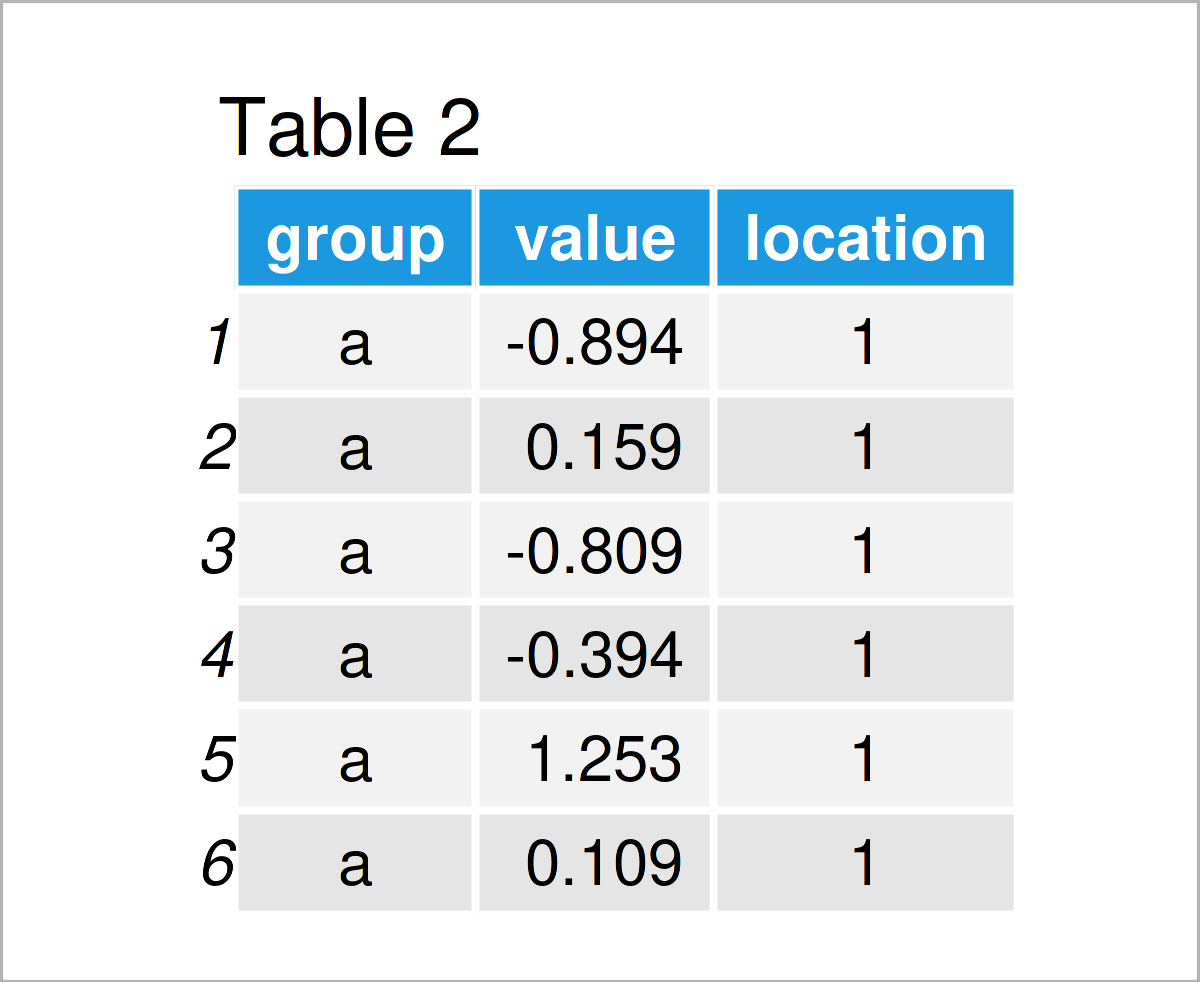# Draw Two ggplot2 Boxplots on Same X-Axis Position in R (Example)

This article demonstrates how to draw multiple boxplots on the same axis location in the R programming language.

Let’s dig in.

## Example Data, Software Packages & Default Graphic

We’ll use the following data as basement for this R programming tutorial:

```set.seed(23732)                       # Create random example data
data <- data.frame(group = rep(letters[1:3], each = 30),
value = c(rnorm(30), rnorm(60, 5)))As you can see based on Table 1, our example data is a data frame containing two columns. The variable group is a character and the variable value is numerical.

For the following tutorial, we’ll also have to install and load the ggplot2 package:

```install.packages("ggplot2")           # Install & load ggplot2
library("ggplot2")```

As a next step, we can draw a graph of our data:

```ggplot(data,                          # ggplot2 boxplot with default positions
aes(x = group,
y = value,
fill = group)) +
geom_boxplot()```By running the previous R programming syntax we have drawn Figure 1, i.e. a ggplot2 boxplot graphic containing three boxes.

## Example: Draw Multiple ggplot2 Boxplots on Same X-Axis Location Using position = “identity”

This example demonstrates how to draw two ggplot2 boxplots on top of each other at the same x-axis position.

For this task, we first have to specify the locations at which we want to draw the different boxes:

```data_loc <- data                      # Duplicate data
data_loc\$location <- c(rep(1, 60),    # Add locations
rep(2, 30))As revealed in Table 2, the previous syntax has created an updated version of our data frame that contains a location column.

In the next step, we can use this new data frame to draw several boxplots at the same axis location.

Note that we are specifying the x argument to be equal to our location column, and we are specifying the position argument within the geom_boxplot function to be equal to “identity”.

```ggplot(data_loc,                      # ggplot2 boxplot with manual locations
aes(x = location,
y = value,
fill = group)) +
geom_boxplot(position = "identity")```By running the previous R code we have created Figure 2, i.e. a ggplot2 boxplot with two boxes at the same x-axis location.

## Video & Further Resources

Have a look at the following video which I have published on my YouTube channel. In the video, I explain the content of this tutorial in a programming session:

Please accept YouTube cookies to play this video. By accepting you will be accessing content from YouTube, a service provided by an external third party.If you accept this notice, your choice will be saved and the page will refresh.

In addition, you may want to have a look at some of the related tutorials on my website.